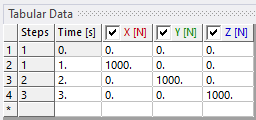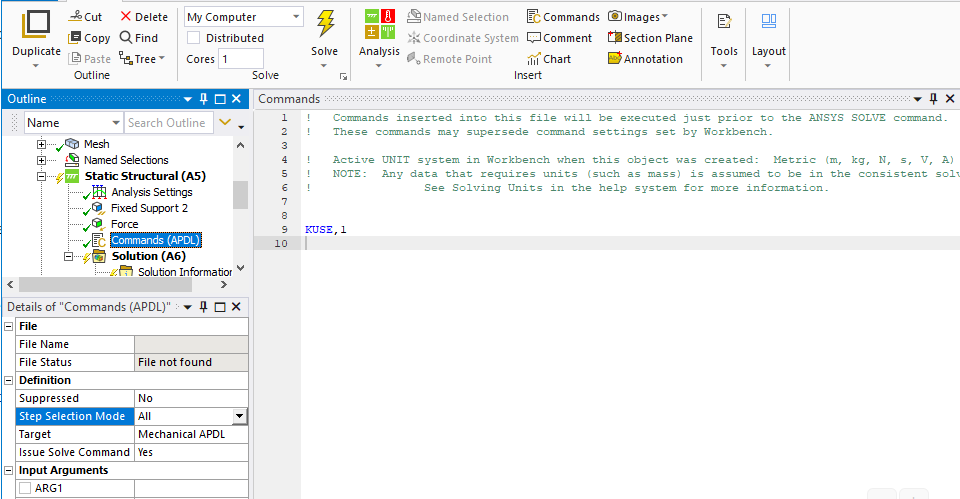peteroznewman
Subscriber
Dear Ahmed,nI am familiar with Femap/Nastran and understand your question perfectly. I asked this same questions when I started using ANSYS. A linear model is a requirement to have what you want, which means: only linear materials, small deflections, only linear contact or no contact.nI have a small linear model that runs within the Student License limits, which is < 32,000 nodes. Under Analysis Settings, I set it for 3 load steps. I have a Force on a face and I put 1000 N in X for Step 1, 1000 N in Y for Step 2 and 1000 N in Z for Step 3.nANSYS uses a lot of program controlled logic to decide what to do, so you don't have to. Deciding whether to reuse the factorized stiffness matrix for the next load step is one of those automatic decisions. There is a way to override that automation and force the solver to reuse the factorized stiffness matrix by inserting a Command into the Static Structural branch of the model in Mechanical. If KUSE is set to 1, the program reuses the previous factorized matrix. KUSE,-1 forces the factorized matrix to be reformulated at every equilibrium iteration or load step.nI set my Solve Process settings to use only 1 core to force the solution time to be as long as possible. nWith no Command object, the solution time is 13 seconds.nWith Command as KUSE, 1 the solution time is 13 seconds.nWith Command as KUSE,-1 the solution time is 21 seconds.nSo you see, you don't have to do anything to get the benefit of reusing the factorized stiffness matrix to compute multiple load case results.nA few years ago, I had a nonlinear model that required a lot of equilibrium iterations in step 1 to pretension some bolts on a flange in nonlinear contact with another part. Step 1 took a few hours to compute. Then I wanted to apply a few different load cases for X, Y and Z. When I put them sequentially, as load steps 2, 3, 4, it took more iterations to finish since the starting point for load step 3 was at the end of load step 2 and the starting point for load step 4 was the end of load step 3. I figured out a way to have each load case start at the end of step 1 and that took fewer iterations. See this discussion. https://forum.ansys.com/discussion/20231/post-processing-in-ansys-mechanical-workbenchnRegards,nPetern